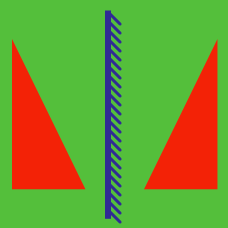Geometry

# Translation

Point $$(2, 1)$$ is moved to point $$(10,11)$$ by $T: (x, y) \rightarrow (x+a, y+b).$ What is $$a+b$$?

If we translate the point $$(9, 3)$$ by $$(13, 2),$$ what would be the new point?

The curve $$y=2x^2+3x-9$$ undergoes a parallel translation by $$7$$ units in the positive direction of the $$x$$-axis and $$-3$$ units in the positive direction of the $$y$$-axis. If the equation of the resulting curve is $$y=ax^2+bx+c,$$ what is $$a+b+c?$$

Consider a translation of axes $T: (x, y) \to (x+a, y+b)$ such that the coordinates of a point $$P=(7, 4)$$ with respect to the new system of coordinates after translation are $$P'=(10,-11).$$ What is the value of $$a+b?$$

If the point $$(m,n)$$ is shifted by $$6$$ in the positive $$x$$ direction and by $$-12$$ in the positive $$y$$ direction, then it coincides with the center of the circle $x^2+y^2-6x+2y-13=0.$ What is the value of $$m+n?$$

×